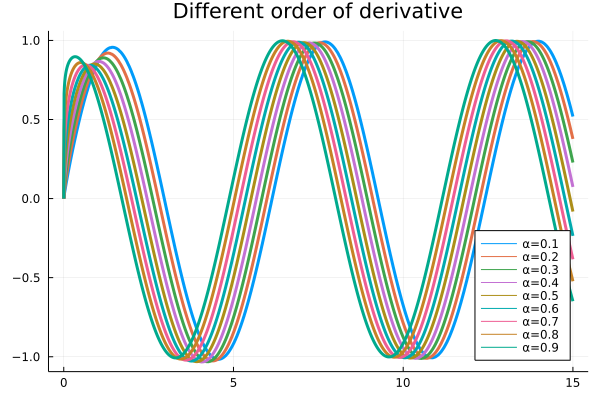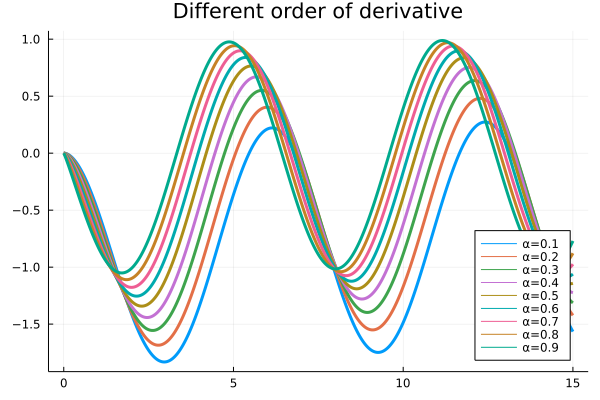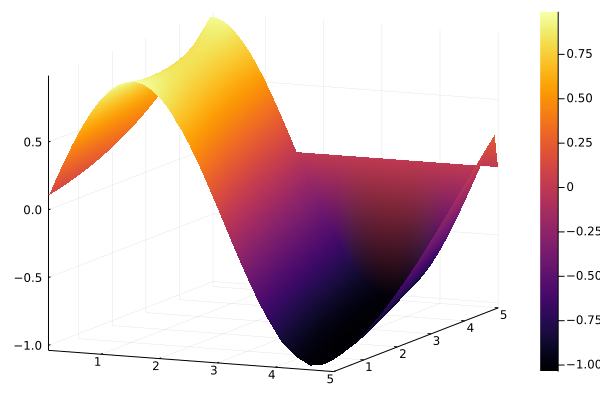# Derivative Example

Let's see how easy it is to compute the fractional derivative of a function🥳

using FractionalCalculus, Plots
tspan=collect(0:0.01:15)
examplederivative = []
for i in range(0.1, 0.9, step=0.1)
push!(examplederivative, fracdiff(sin, i, tspan, 0.001, RLDiff_Approx()))
end
plot(tspan, examplederivative, title="Different order of derivative", linewidth=3, label=["α=0.1" "α=0.2" "α=0.3" "α=0.4" "α=0.5" "α=0.6" "α=0.7" "α=0.8" "α=0.9" "α=1"], legend=:bottomright)

Here, we use Plots.jl to generate the computing result:Or different order derivative of $f(x)=cos(x)$:

using FractionalCalculus, Plots
tspan=collect(0:0.01:15)
examplederivative = []
for i in range(0.1, 0.9, step=0.1)
push!(examplederivative, fracdiff(cos, i, tspan, 0.001, CaputoDiethelm()))
end
plot(tspan, examplederivative, title="Different order of derivative", linewidth=3, label=["α=0.1" "α=0.2" "α=0.3" "α=0.4" "α=0.5" "α=0.6" "α=0.7" "α=0.8" "α=0.9" "α=1"], legend=:bottomright)Also want to see different order derivative of a function? No problem!!